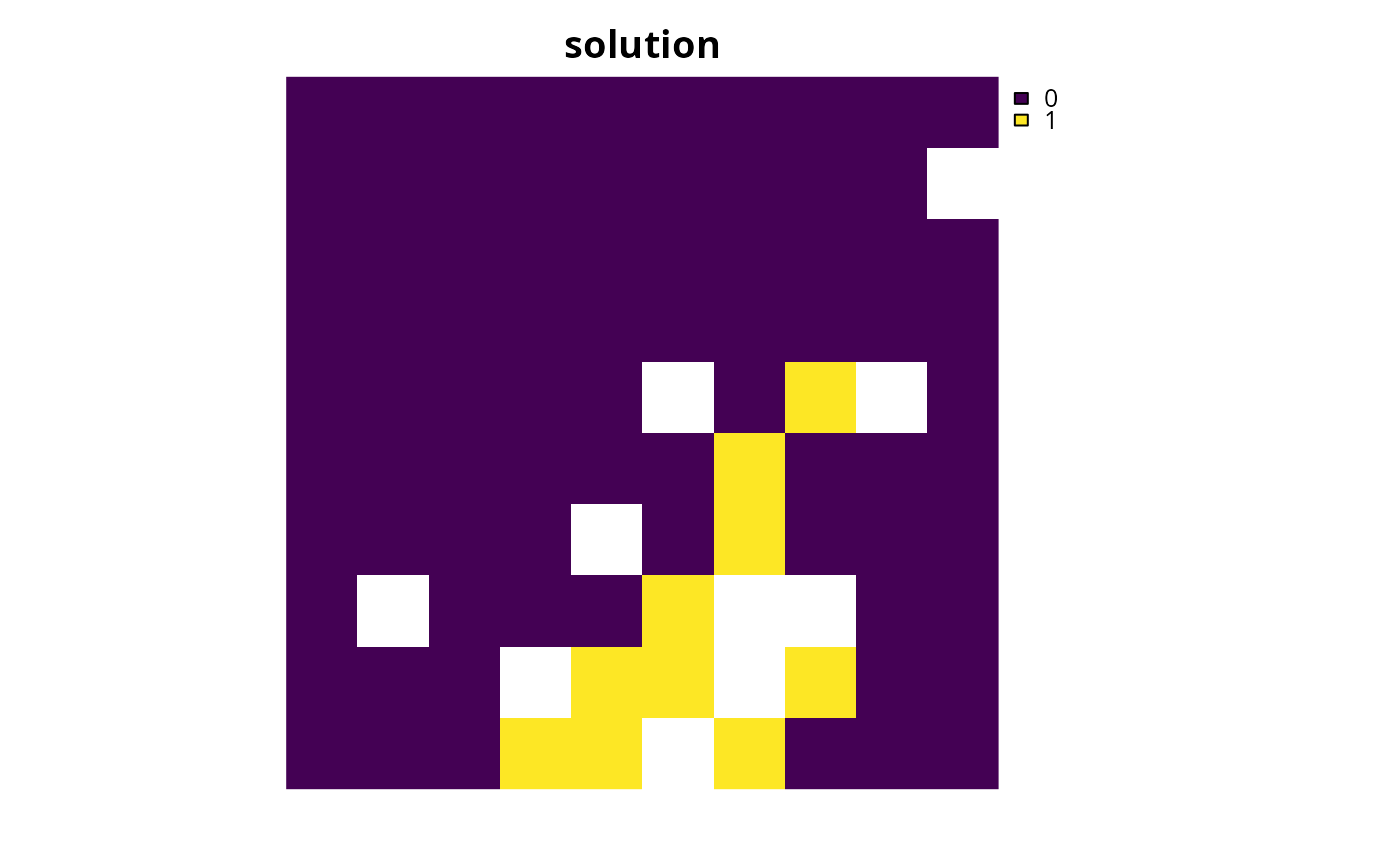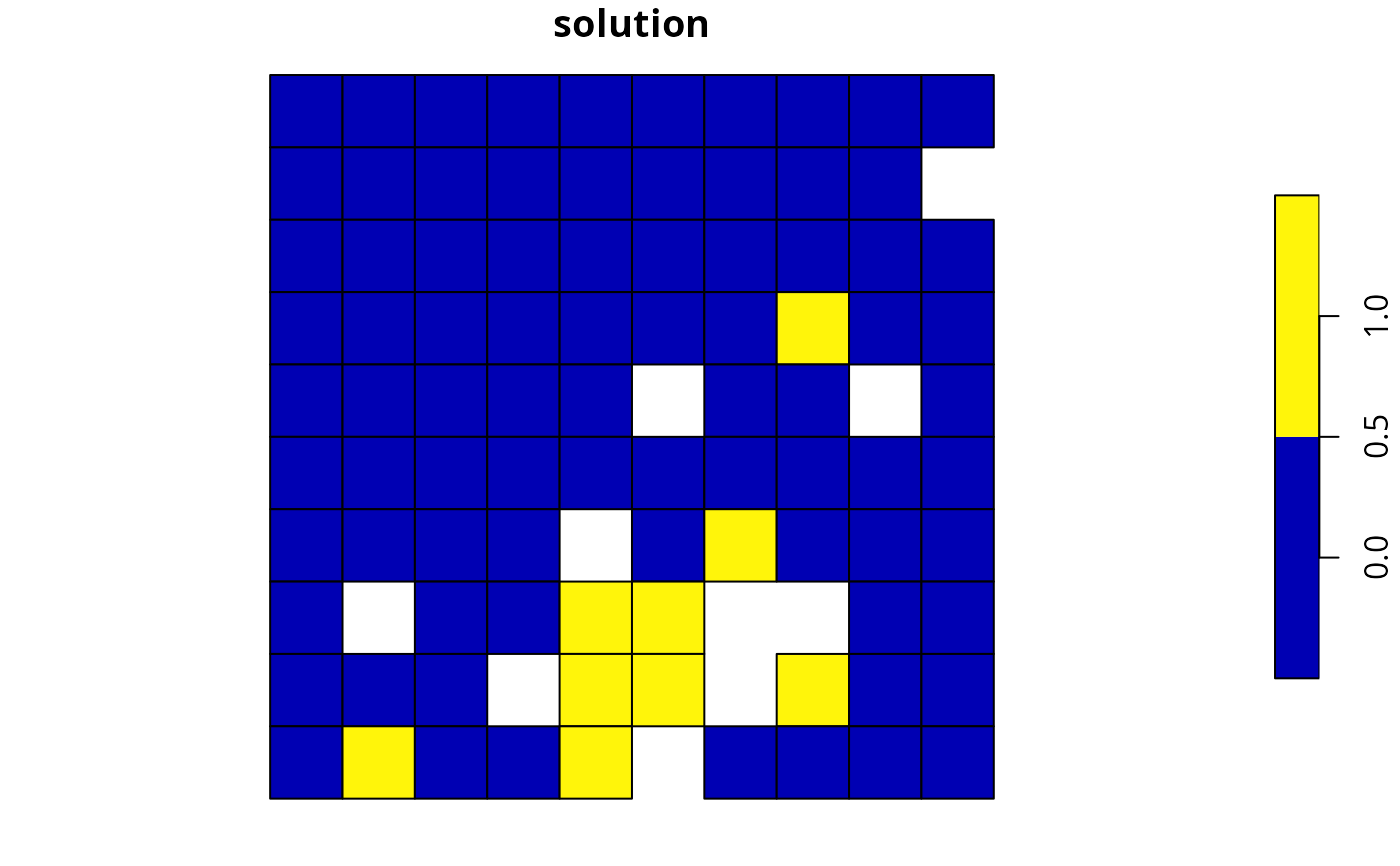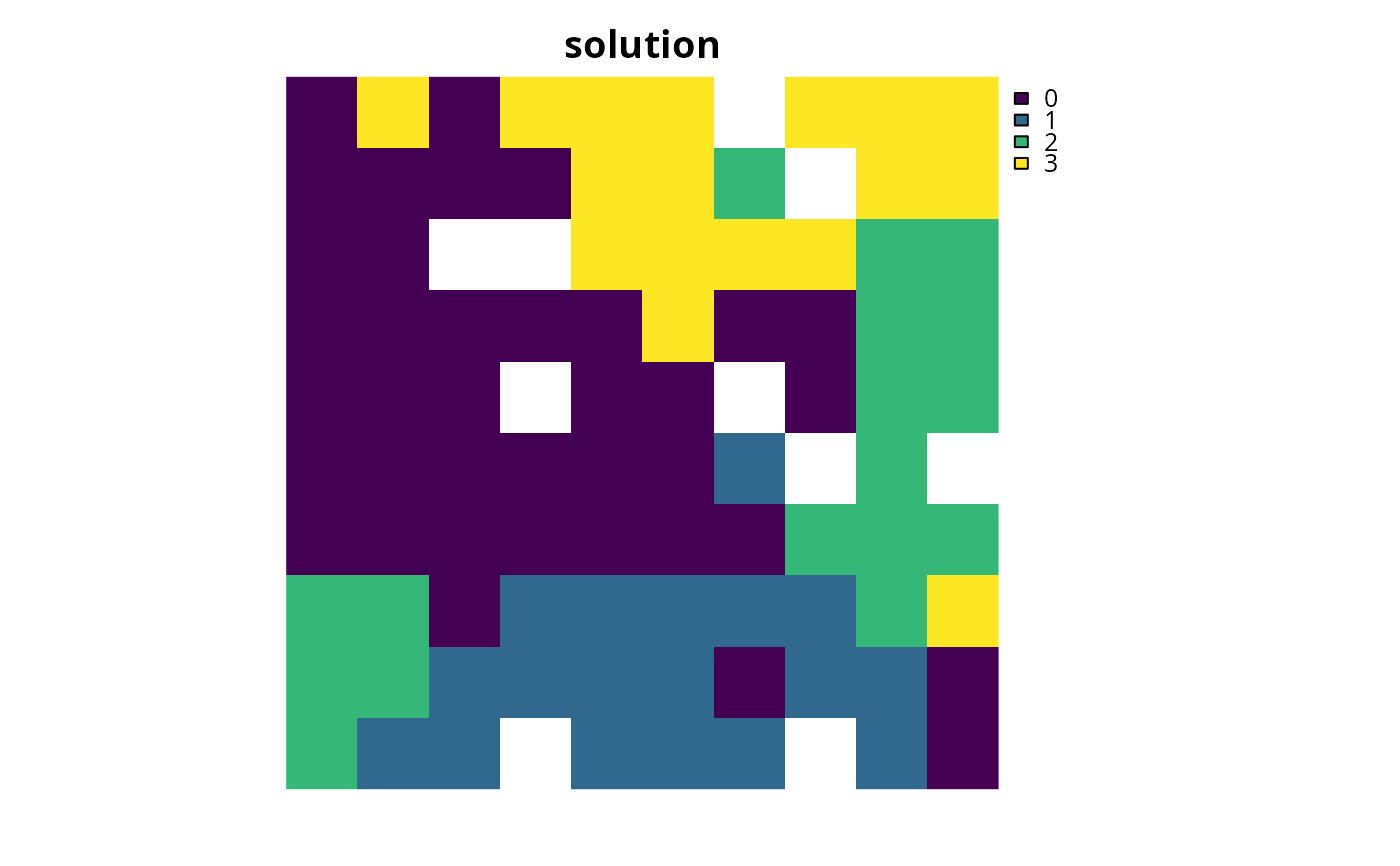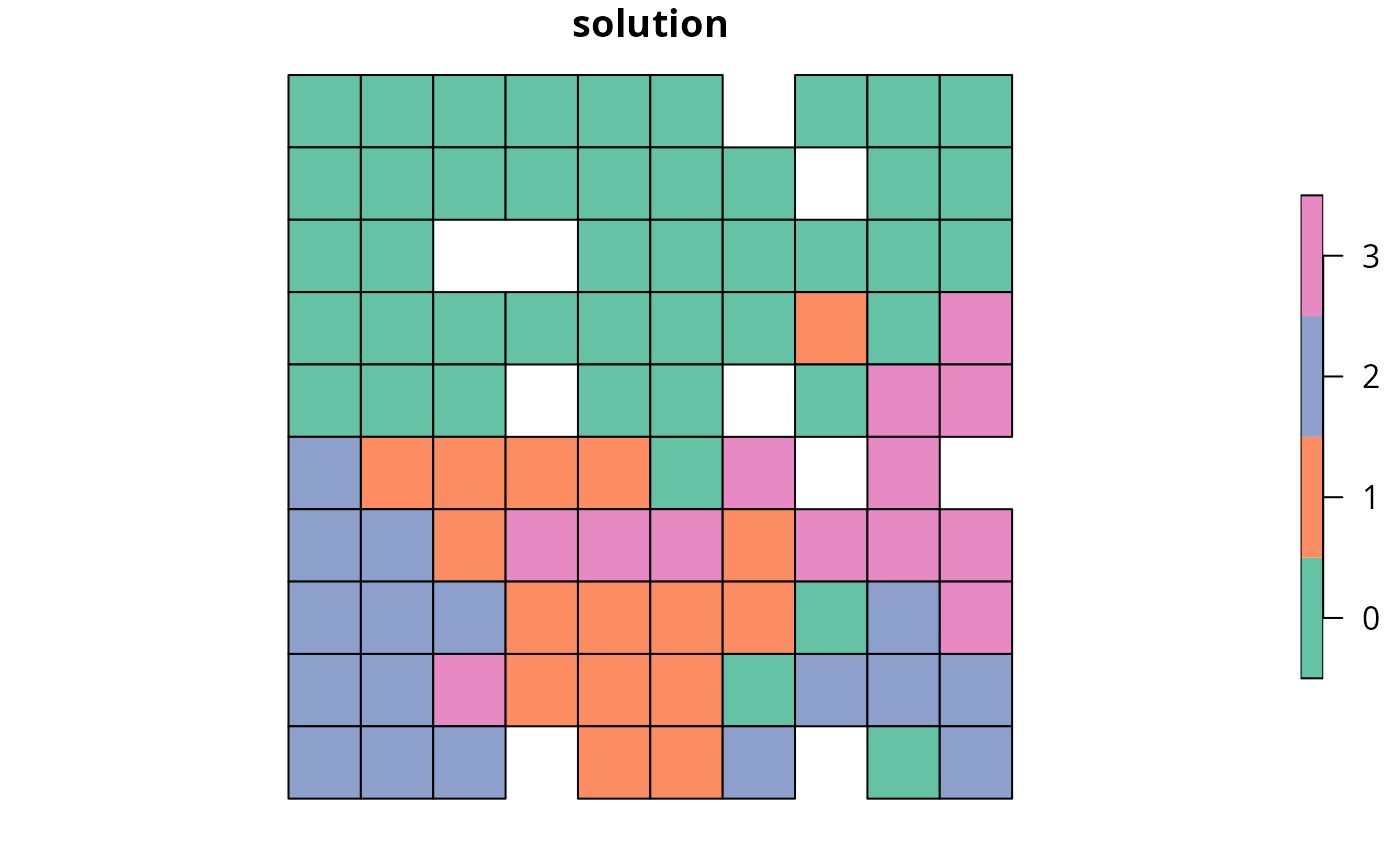Calculate how well features are represented by a solution to a conservation planning problem(). These summary statistics are reported for each and every feature, and each and every zone, within a conservation planning problem.

eval_feature_representation_summary(x, solution)

# S4 method for ConservationProblem,numeric
eval_feature_representation_summary(x, solution)

# S4 method for ConservationProblem,matrix
eval_feature_representation_summary(x, solution)

# S4 method for ConservationProblem,data.frame
eval_feature_representation_summary(x, solution)

# S4 method for ConservationProblem,Spatial
eval_feature_representation_summary(x, solution)

# S4 method for ConservationProblem,sf
eval_feature_representation_summary(x, solution)

# S4 method for ConservationProblem,Raster
eval_feature_representation_summary(x, solution)

## Arguments

x

problem() (i.e., ConservationProblem) object.

solution

numeric, matrix, data.frame, Raster, Spatial, or sf::sf() object. The argument should be in the same format as the planning unit cost data in the argument to x. See the Solution format section for more information.

## Value

tibble::tibble() object describing feature representation. Here, each row describes a specific summary statistic (e.g., different management zone) for a specific feature. It contains the following columns:

summary

character description of the summary statistic. The statistic associated with the "overall" value in this column is calculated using the entire solution (including all management zones if there are multiple zones). If multiple management zones are present, then summary statistics are also provided for each zone separately (indicated using zone names).

feature

character name of the feature.

total_amount

numeric total amount of each feature available in the entire conservation planning problem (not just planning units selected within the solution). It is calculated as the sum of the feature data, supplied when creating a problem() object (e.g., presence/absence values).

absolute_held

numeric total amount of each feature secured within the solution. It is calculated as the sum of the feature data, supplied when creating a problem() object (e.g., presence/absence values), weighted by the status of each planning unit in the solution (e.g., selected or not for prioritization).

relative_held

numeric proportion of each feature secured within the solution. It is calculated by dividing values in the "absolute_held" column by those in the "total_amount" column.

## Solution format

Broadly speaking, the argument to solution must be in the same format as the planning unit data in the argument to x. Further details on the correct format are listed separately for each of the different planning unit data formats:

x has numeric planning units

The argument to solution must be a numeric vector with each element corresponding to a different planning unit. It should have the same number of planning units as those in the argument to x. Additionally, any planning units missing cost (NA) values should also have missing (NA) values in the argument to solution.

x has matrix planning units

The argument to solution must be a matrix vector with each row corresponding to a different planning unit, and each column correspond to a different management zone. It should have the same number of planning units and zones as those in the argument to x. Additionally, any planning units missing cost (NA) values for a particular zone should also have a missing (NA) values in the argument to solution.

x has Raster planning units

The argument to solution be a Raster object where different grid cells (pixels) correspond to different planning units and layers correspond to a different management zones. It should have the same dimensionality (rows, columns, layers), resolution, extent, and coordinate reference system as the planning units in the argument to x. Additionally, any planning units missing cost (NA) values for a particular zone should also have missing (NA) values in the argument to solution.

x has data.frame planning units

The argument to solution must be a data.frame with each column corresponding to a different zone, each row corresponding to a different planning unit, and cell values corresponding to the solution value. This means that if a data.frame object containing the solution also contains additional columns, then these columns will need to be subsetted prior to using this function (see below for example with sf::sf() data). Additionally, any planning units missing cost (NA) values for a particular zone should also have missing (NA) values in the argument to solution.

x has Spatial planning units

The argument to solution must be a Spatial object with each column corresponding to a different zone, each row corresponding to a different planning unit, and cell values corresponding to the solution value. This means that if the Spatial object containing the solution also contains additional columns, then these columns will need to be subsetted prior to using this function (see below for example with sf::sf() data). Additionally, the argument to solution must also have the same coordinate reference system as the planning unit data. Furthermore, any planning units missing cost (NA) values for a particular zone should also have missing (NA) values in the argument to solution.

x has sf::sf() planning units

The argument to solution must be a sf::sf() object with each column corresponding to a different zone, each row corresponding to a different planning unit, and cell values corresponding to the solution value. This means that if the sf::sf() object containing the solution also contains additional columns, then these columns will need to be subsetted prior to using this function (see below for example). Additionally, the argument to solution must also have the same coordinate reference system as the planning unit data. Furthermore, any planning units missing cost (NA) values for a particular zone should also have missing (NA) values in the argument to solution.

See summaries for an overview of all functions for summarizing solutions.

Other summaries: eval_asym_connectivity_summary(), eval_boundary_summary(), eval_connectivity_summary(), eval_cost_summary(), eval_n_summary(), eval_target_coverage_summary()

## Examples

# \dontrun{
# set seed for reproducibility
set.seed(500)

data(sim_pu_raster, sim_pu_polygons, sim_pu_zones_sf, sim_features,
sim_pu_zones_stack, sim_features_zones)

# create a simple conservation planning dataset so we can see exactly
# how feature representation is calculated
pu <- data.frame(id = seq_len(10), cost = c(0.2, NA, runif(8)),
spp1 = runif(10), spp2 = c(rpois(9, 4), NA))

# create problem
p1 <- problem(pu, c("spp1", "spp2"), cost_column = "cost") %>%

# create a solution
# specifically, a data.frame with a single column that contains
# binary values indicating if each planning units was selected or not
s1 <- data.frame(s = c(1, NA, rep(c(1, 0), 4)))
print(s1)
#>     s
#> 1   1
#> 2  NA
#> 3   1
#> 4   0
#> 5   1
#> 6   0
#> 7   1
#> 8   0
#> 9   1
#> 10  0

# calculate feature representation
r1 <- eval_feature_representation_summary(p1, s1)
print(r1)
#> # A tibble: 2 × 5
#>   summary feature total_amount absolute_held relative_held
#>   <chr>   <chr>          <dbl>         <dbl>         <dbl>
#> 1 overall spp1            5.76          3.12         0.541
#> 2 overall spp2           33            14            0.424

# let's verify that feature representation calculations are correct
# by manually performing the calculations and compare the results with r1
## calculate total amount for each feature
print(setNames(
c(sum(pu$spp1, na.rm = TRUE), sum(pu$spp2, na.rm = TRUE)),
c("spp1", "spp2")))
#>      spp1      spp2
#>  5.755739 33.000000

## calculate absolute amount held for each feature
print(setNames(
c(sum(pu$spp1 * s1$s, na.rm = TRUE),
sum(pu$spp2 * s1$s, na.rm = TRUE)),
c("spp1", "spp2")))
#>      spp1      spp2
#>  3.116052 14.000000

## calculate relative amount held for each feature
print(setNames(
c(sum(pu$spp1 * s1$s, na.rm = TRUE) / sum(pu$spp1, na.rm = TRUE), sum(pu$spp2 * s1$s, na.rm = TRUE) / sum(pu$spp2, na.rm = TRUE)),
c("spp1", "spp2")))
#>      spp1      spp2
#> 0.5413818 0.4242424

# solve the problem using an exact algorithm solver
s1_2 <- solve(p1)
print(s1_2)
#>    id      cost      spp1 spp2 solution_1
#> 1   1 0.2000000 0.8288314    4          1
#> 2   2        NA 0.7115770    3         NA
#> 3   3 0.8336000 0.2820609    1          0
#> 4   4 0.7250118 0.8928427    6          0
#> 5   5 0.9753142 0.7647062    1          0
#> 6   6 0.4676038 0.1643470    4          0
#> 7   7 0.8122781 0.7320744    3          0
#> 8   8 0.2056958 0.2531062    6          0
#> 9   9 0.5121819 0.5083795    5          0
#> 10 10 0.9254660 0.6178138   NA          0

# calculate feature representation in this solution
r1_2 <- eval_feature_representation_summary(
p1, s1_2[, "solution_1", drop = FALSE])
print(r1_2)
#> # A tibble: 2 × 5
#>   summary feature total_amount absolute_held relative_held
#>   <chr>   <chr>          <dbl>         <dbl>         <dbl>
#> 1 overall spp1            5.76         0.829         0.144
#> 2 overall spp2           33            4             0.121

# build minimal conservation problem with raster data
p2 <- problem(sim_pu_raster, sim_features) %>%

# solve the problem
s2 <- solve(p2)

# print solution
print(s2)
#> class      : RasterLayer
#> dimensions : 10, 10, 100  (nrow, ncol, ncell)
#> resolution : 0.1, 0.1  (x, y)
#> extent     : 0, 1, 0, 1  (xmin, xmax, ymin, ymax)
#> crs        : NA
#> source     : memory
#> names      : layer
#> values     : 0, 1  (min, max)
#>

# calculate feature representation in the solution
r2 <- eval_feature_representation_summary(p2, s2)
print(r2)
#> # A tibble: 5 × 5
#>   summary feature total_amount absolute_held relative_held
#>   <chr>   <chr>          <dbl>         <dbl>         <dbl>
#> 1 overall layer.1         83.3          8.91         0.107
#> 2 overall layer.2         31.2          3.13         0.100
#> 3 overall layer.3         72.0          7.34         0.102
#> 4 overall layer.4         42.7          4.35         0.102
#> 5 overall layer.5         56.7          6.01         0.106

# plot solution
plot(s2, main = "solution", axes = FALSE, box = FALSE)# build minimal conservation problem with polygon (Spatial) data
p3 <- problem(sim_pu_polygons, sim_features, cost_column = "cost") %>%

# solve the problem
s3 <- solve(p3)

# print first six rows of the attribute table
#>       cost locked_in locked_out solution_1
#> 1 215.8638     FALSE      FALSE          0
#> 2 212.7823     FALSE      FALSE          0
#> 3 207.4962     FALSE      FALSE          0
#> 4 208.9322     FALSE       TRUE          0
#> 5 214.0419     FALSE      FALSE          0
#> 6 213.7636     FALSE      FALSE          0

# calculate feature representation in the solution
r3 <- eval_feature_representation_summary(p3, s3[, "solution_1"])
print(r3)
#> # A tibble: 5 × 5
#>   summary feature total_amount absolute_held relative_held
#>   <chr>   <chr>          <dbl>         <dbl>         <dbl>
#> 1 overall layer.1         74.5          8.05         0.108
#> 2 overall layer.2         28.1          2.83         0.101
#> 3 overall layer.3         64.9          6.65         0.103
#> 4 overall layer.4         38.2          3.87         0.101
#> 5 overall layer.5         50.7          5.41         0.107

# plot solution
spplot(s3, zcol = "solution_1", main = "solution", axes = FALSE, box = FALSE)# build multi-zone conservation problem with raster data
p4 <- problem(sim_pu_zones_stack, sim_features_zones) %>%
add_relative_targets(matrix(runif(15, 0.1, 0.2), nrow = 5,
ncol = 3)) %>%

# solve the problem
s4 <- solve(p4)

# print solution
print(s4)
#> class      : RasterStack
#> dimensions : 10, 10, 100, 3  (nrow, ncol, ncell, nlayers)
#> resolution : 0.1, 0.1  (x, y)
#> extent     : 0, 1, 0, 1  (xmin, xmax, ymin, ymax)
#> crs        : NA
#> names      : zone_1, zone_2, zone_3
#> min values :      0,      0,      0
#> max values :      1,      1,      1
#>

# calculate feature representation in the solution
r4 <- eval_feature_representation_summary(p4, s4)
print(r4)
#> # A tibble: 20 × 5
#>    summary feature   total_amount absolute_held relative_held
#>    <chr>   <chr>            <dbl>         <dbl>         <dbl>
#>  1 overall feature_1        250.          45.8          0.183
#>  2 overall feature_2         93.6         17.7          0.189
#>  3 overall feature_3        216.          40.2          0.186
#>  4 overall feature_4        128.          23.7          0.186
#>  5 overall feature_5        170.          31.2          0.183
#>  6 zone_1  feature_1         83.3         16.0          0.192
#>  7 zone_1  feature_2         31.2          5.07         0.162
#>  8 zone_1  feature_3         72.0         13.2          0.183
#>  9 zone_1  feature_4         42.7          7.04         0.165
#> 10 zone_1  feature_5         56.7         11.2          0.197
#> 11 zone_2  feature_1         83.3         16.0          0.192
#> 12 zone_2  feature_2         31.2          6.45         0.207
#> 13 zone_2  feature_3         72.0         15.1          0.210
#> 14 zone_2  feature_4         42.7          8.22         0.193
#> 15 zone_2  feature_5         56.7         10.4          0.184
#> 16 zone_3  feature_1         83.3         13.8          0.166
#> 17 zone_3  feature_2         31.2          6.16         0.197
#> 18 zone_3  feature_3         72.0         11.9          0.165
#> 19 zone_3  feature_4         42.7          8.49         0.199
#> 20 zone_3  feature_5         56.7          9.58         0.169

# plot solution
plot(category_layer(s4), main = "solution", axes = FALSE, box = FALSE)# build multi-zone conservation problem with polygon (sf) data
p5 <- problem(sim_pu_zones_sf, sim_features_zones,
cost_column = c("cost_1", "cost_2", "cost_3")) %>%
add_relative_targets(matrix(runif(15, 0.1, 0.2), nrow = 5,
ncol = 3)) %>%

# solve the problem
s5 <- solve(p5)

# print first six rows of the attribute table
#> Simple feature collection with 6 features and 9 fields
#> Geometry type: POLYGON
#> Dimension:     XY
#> Bounding box:  xmin: 0 ymin: 0.9 xmax: 0.6 ymax: 1
#> CRS:           NA
#>     cost_1   cost_2   cost_3 locked_1 locked_2 locked_3 solution_1_zone_1
#> 1 215.8638 183.3344 205.4113    FALSE    FALSE    FALSE                 0
#> 2 212.7823 189.4978 209.6404    FALSE    FALSE    FALSE                 0
#> 3 207.4962 193.6007 215.4212     TRUE    FALSE    FALSE                 0
#> 4 208.9322 197.5897 218.5241    FALSE    FALSE    FALSE                 0
#> 5 214.0419 199.8033 220.7100    FALSE    FALSE    FALSE                 0
#> 6 213.7636 203.1867 224.6809    FALSE    FALSE    FALSE                 0
#>   solution_1_zone_2 solution_1_zone_3                       geometry
#> 1                 1                 0 POLYGON ((0 1, 0.1 1, 0.1 0...
#> 2                 0                 0 POLYGON ((0.1 1, 0.2 1, 0.2...
#> 3                 0                 0 POLYGON ((0.2 1, 0.3 1, 0.3...
#> 4                 0                 0 POLYGON ((0.3 1, 0.4 1, 0.4...
#> 5                 0                 0 POLYGON ((0.4 1, 0.5 1, 0.5...
#> 6                 0                 0 POLYGON ((0.5 1, 0.6 1, 0.6...

# calculate feature representation in the solution
r5 <- eval_feature_representation_summary(
p5, s5[, c("solution_1_zone_1", "solution_1_zone_2", "solution_1_zone_3")])
print(r5)
#> # A tibble: 20 × 5
#>    summary feature   total_amount absolute_held relative_held
#>    <chr>   <chr>            <dbl>         <dbl>         <dbl>
#>  1 overall feature_1        225.          40.9          0.182
#>  2 overall feature_2         83.9         13.8          0.164
#>  3 overall feature_3        195.          34.3          0.176
#>  4 overall feature_4        114.          18.7          0.164
#>  5 overall feature_5        154.          28.3          0.184
#>  6 zone_1  feature_1         75.1         14.9          0.198
#>  7 zone_1  feature_2         28.0          4.77         0.170
#>  8 zone_1  feature_3         65.0         12.7          0.195
#>  9 zone_1  feature_4         38.0          6.39         0.168
#> 10 zone_1  feature_5         51.2         10.2          0.200
#> 11 zone_2  feature_1         75.1         15.0          0.199
#> 12 zone_2  feature_2         28.0          5.02         0.179
#> 13 zone_2  feature_3         65.0         12.9          0.199
#> 14 zone_2  feature_4         38.0          6.03         0.158
#> 15 zone_2  feature_5         51.2         10.2          0.199
#> 16 zone_3  feature_1         75.1         11.1          0.148
#> 17 zone_3  feature_2         28.0          3.98         0.142
#> 18 zone_3  feature_3         65.0          8.71         0.134
#> 19 zone_3  feature_4         38.0          6.33         0.166
#> 20 zone_3  feature_5         51.2          7.84         0.153

# create new column representing the zone id that each planning unit
# was allocated to in the solution
s5$solution <- category_vector( s5[, c("solution_1_zone_1", "solution_1_zone_2", "solution_1_zone_3")]) s5$solution <- factor(s5\$solution)

# plot solution
plot(s5[, "solution"])# }